# Data Mining Data Lecture Notes for Chapter 2

• Slides: 15Data Mining: Data Lecture Notes for Chapter 2 Introduction to PCA (Principal Component Analysis) © Tan, Steinbach, Kumar Introduction to Data Mining 4/18/2004 1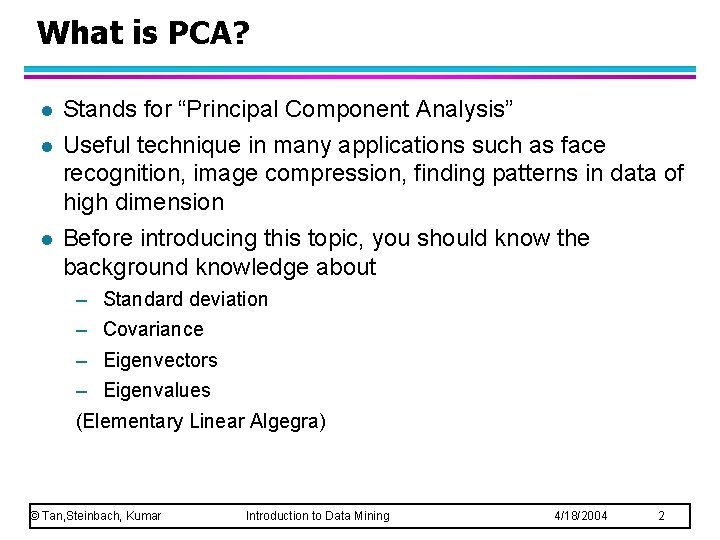What is PCA? l l l Stands for “Principal Component Analysis” Useful technique in many applications such as face recognition, image compression, finding patterns in data of high dimension Before introducing this topic, you should know the background knowledge about – Standard deviation – Covariance – Eigenvectors – Eigenvalues (Elementary Linear Algegra) © Tan, Steinbach, Kumar Introduction to Data Mining 4/18/2004 2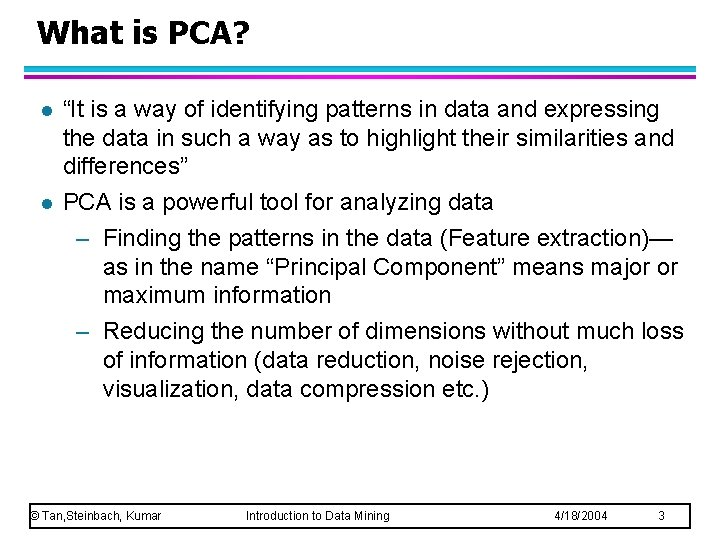What is PCA? l l “It is a way of identifying patterns in data and expressing the data in such a way as to highlight their similarities and differences” PCA is a powerful tool for analyzing data – Finding the patterns in the data (Feature extraction)— as in the name “Principal Component” means major or maximum information – Reducing the number of dimensions without much loss of information (data reduction, noise rejection, visualization, data compression etc. ) © Tan, Steinbach, Kumar Introduction to Data Mining 4/18/2004 3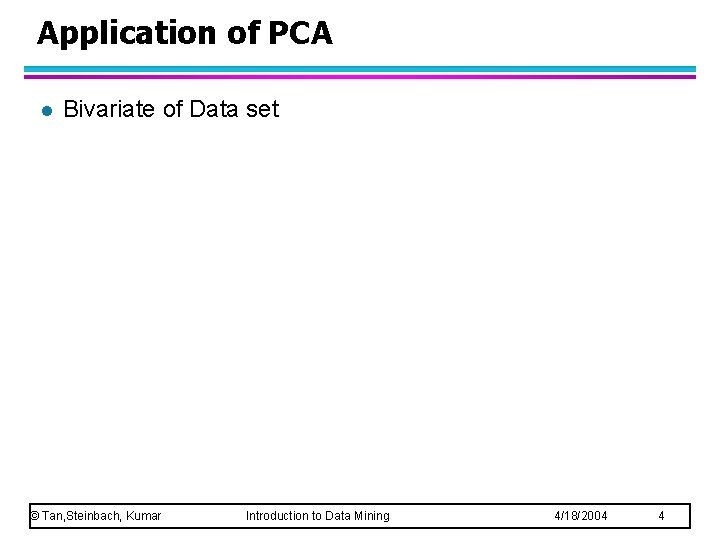Application of PCA l Bivariate of Data set © Tan, Steinbach, Kumar Introduction to Data Mining 4/18/2004 4Tutorial by Example l Step 1: Get some data © Tan, Steinbach, Kumar Introduction to Data Mining 4/18/2004 5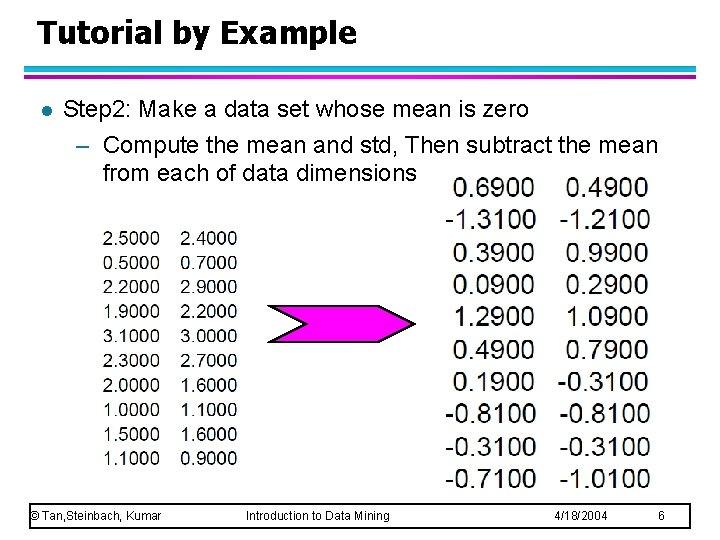Tutorial by Example l Step 2: Make a data set whose mean is zero – Compute the mean and std, Then subtract the mean from each of data dimensions © Tan, Steinbach, Kumar Introduction to Data Mining 4/18/2004 6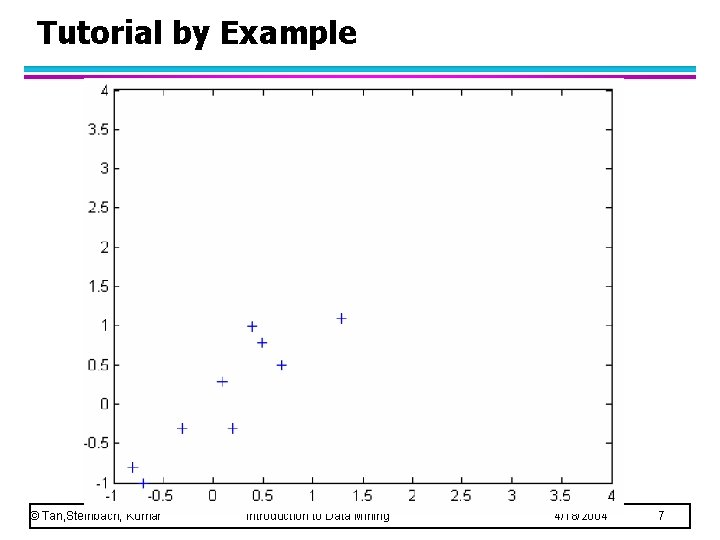Tutorial by Example © Tan, Steinbach, Kumar Introduction to Data Mining 4/18/2004 7Tutorial by Example Step 3: Calculate the covariance matrix (see PCATutorial. pdf) l Since the data is 2 dim, the covariance matrix will be 2 x 2 l What to notice? © Tan, Steinbach, Kumar Introduction to Data Mining 4/18/2004 8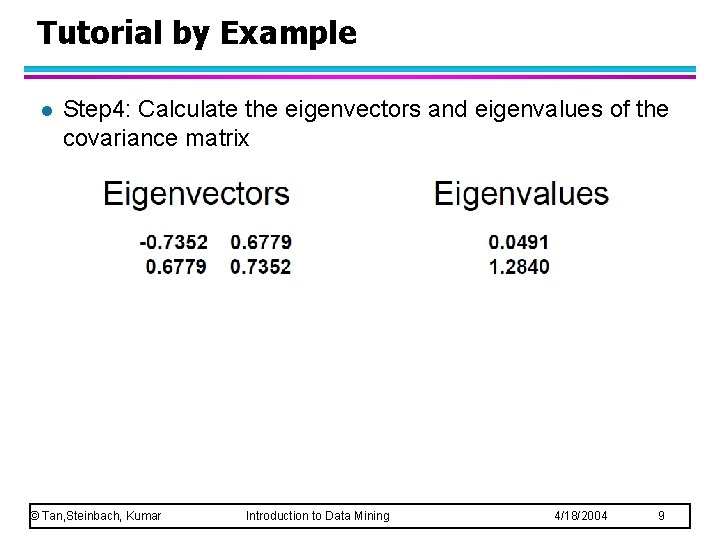Tutorial by Example l Step 4: Calculate the eigenvectors and eigenvalues of the covariance matrix © Tan, Steinbach, Kumar Introduction to Data Mining 4/18/2004 9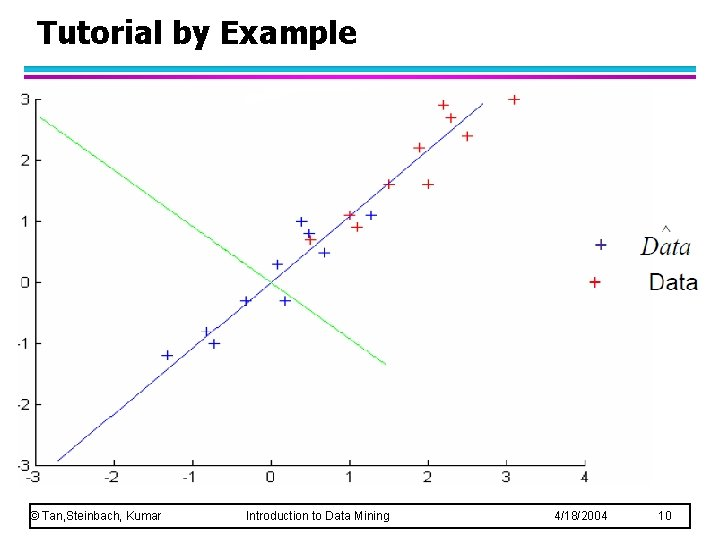Tutorial by Example © Tan, Steinbach, Kumar Introduction to Data Mining 4/18/2004 10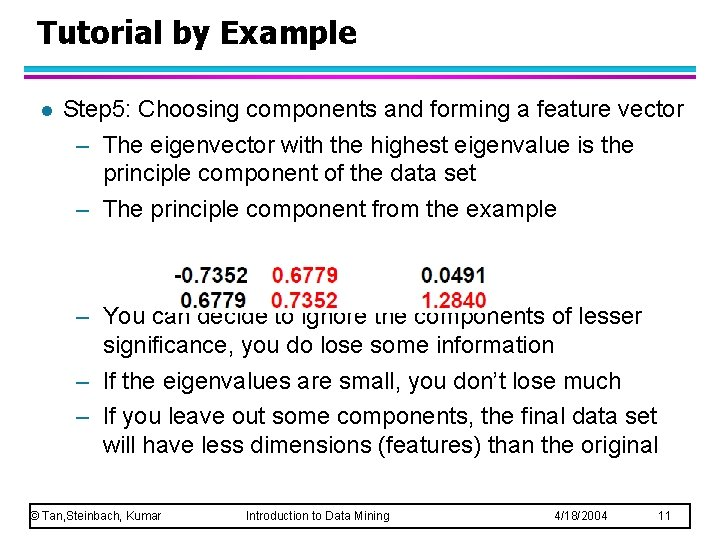Tutorial by Example l Step 5: Choosing components and forming a feature vector – The eigenvector with the highest eigenvalue is the principle component of the data set – The principle component from the example – You can decide to ignore the components of lesser significance, you do lose some information – If the eigenvalues are small, you don’t lose much – If you leave out some components, the final data set will have less dimensions (features) than the original © Tan, Steinbach, Kumar Introduction to Data Mining 4/18/2004 11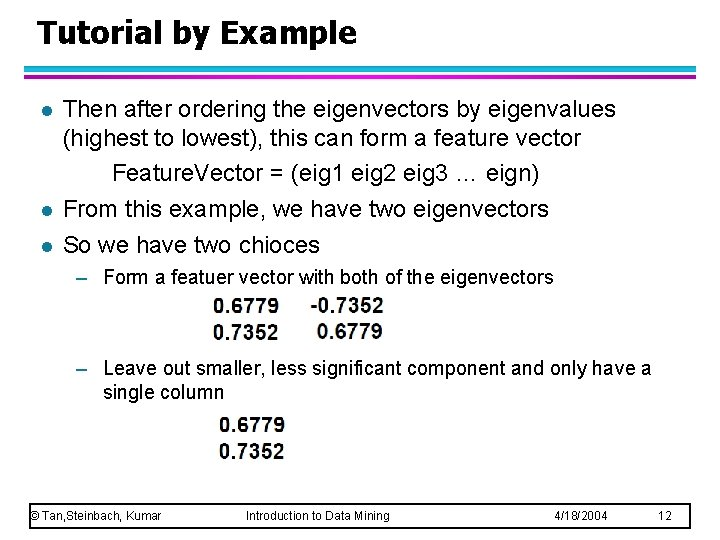Tutorial by Example l l l Then after ordering the eigenvectors by eigenvalues (highest to lowest), this can form a feature vector Feature. Vector = (eig 1 eig 2 eig 3 … eign) From this example, we have two eigenvectors So we have two chioces – Form a featuer vector with both of the eigenvectors – Leave out smaller, less significant component and only have a single column © Tan, Steinbach, Kumar Introduction to Data Mining 4/18/2004 12Tutorial by Example l Step 6 : Deriving the new data set © Tan, Steinbach, Kumar Introduction to Data Mining 4/18/2004 13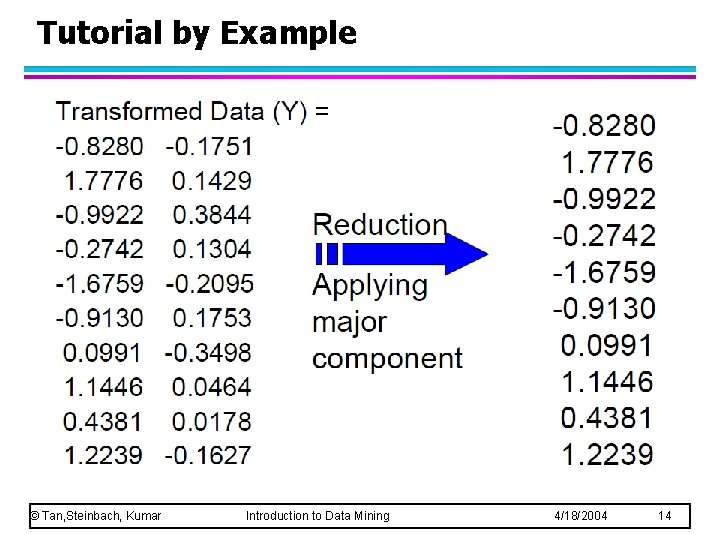Tutorial by Example © Tan, Steinbach, Kumar Introduction to Data Mining 4/18/2004 14Tutorial by Example © Tan, Steinbach, Kumar Introduction to Data Mining 4/18/2004 15